# Clustering Maps¶

Within each of the neighborhoods, there are several versions of each map. In this analysis, we'll cluster version of each of the maps, based on the variations of the maps and the size of the region formed by the four ground control point intersections.

For instance, here are two versions of the East Village map.Part of the process for digitizing these maps is to annotate four well-known intersections on each map; these intersection positions are known as ground control points. These annotations are used to transform pixel position to geographic coordinates, but they can also be used to determine the scale for a map, but looking at the four positions as a rectangle. Here is the intersection polygon for the East Village Maps.We can use the (x,y) coordinates for these rectangles to do a k-means clustering on the maps, and later use the map grouping and scale information as input to extracting features that can help identify similar maps in the future.

In :
import seaborn as sns
import metapack as mp
import pandas as pd
import numpy as np
import matplotlib.pyplot as plt
from IPython.display import display
from kneed import KneeLocator
from shapely import wkt
import dtcv
from dtcv.images import get_image, upper_left
from dtcv.plotting import *
from sklearn.cluster import KMeans
import statsmodels.api as sm
import cv2

base_cmap = plt.get_cmap('gist_rainbow')

%matplotlib inline
sns.set_context('notebook')
mp.jupyter.init()


# Load in the CV Datasets¶

First, load in the Downtown Homelessness Computer Vision datasets.

In :
pkg = mp.jupyter.open_package()
#pkg = mp.jupyter.open_source_package()
pkg

Out:

# San Diego Downtown Homless Computer Vision Package

sandiegodata.org-downtown_cv-4 Last Update: 2019-09-13T01:02:13

Files and code for analyzing San Diego downtown homelessness data with computer vision

This dataset collects records related to a conversion of 5 years of paper maps that record positions of homeless sleepers in downtown San Diego. The San Diego Regional Data Library is converting these paper maps to a digital form with a manual process that uses an image annotation tool, and theses annotations can be used to train computer vision algorithms to georeference maps and recognize handwritten marks.

These datasets link to map urls and annotations, for three kinds of annotations:

• Ground Control Points, which identify the map image locations for known intersections, linking image coordinates ( in pixels ) to geographic coordinates.
• Image locations of handwritten marks and the number written in the mark.
• File annotations, for other handwritten notes such as the temperature and presence of rain.

For more discussion about the GCP and handwritten marks, and the tasks in volved in developing computer vision algorithms for these data, see our recent blog post on the subject.

For some examples of using OpenCV to extract and match templates, to georeference maps, see the Templates and Clustering Jupyter Notebook

## Developer notes

After anotation JSON files are copied into S#, the list of S# urls must be updated. To refresh the list of urls run

\$  bin/update_s3.sh <s3-profile>


## Resources

The intersection_regions dataset has URLS for each of the map images, as well as Shapely ( WKT format ) polygons composed of the intersection points. The gcp dataset has the individual, labeld intersections, but for our purposes, the polygons are easier to use.

In :
r = pkg.resource('intersection_regions')
df = r.dataframe()
display(r)


### intersection_regions

file:///Users/eric/proj/data-projects/homelessness/datasets/sandiegodata.org-downtown_cv/_packages/sandiegodata.org-downtown_cv-4/data/intersection_regions.csv

image_urlstringUrl to a map image
neighborhoodstringName of the neighborhood for the maps
yearintegerYear portion of the data collection date.
monthintegerMonth portion of the data collection date.
intersections_idstringA string composed of the names of the four intersections.
intersection_groupstringA name, based on the neighbrhood, that identifies distinct intersection_id strings.
map_namestringA name based on the neighborhood and map changes in 2016 and 2017
source_invstringThe intersection polygon, fromed from the intersection points, in WKT format, in the pixel coordinate space. This version is inverted, with the Y coordinate being subtracted from 2000, so the orientation of the Y axis is the same as the EPSG:2230 geographic coordinate space.
sourcestringLike source_inv, but the Y axis is not inverted, so the coordinates are the same as the image.
source_areanumberArea of source shape, in square pixels
source_shapestring(X,Y) shape of source polygon bounding box
source_shape_xintegerX value of source_shape
source_shape_yintegerY value of source_shape
deststringThe intersection polygon, but in EPSG:2230 (State plane 6, California, Feet) coordinates.
matrixstringAn affine transformation matric that transforms from the coorinates of source_inv to dest. When pixel locations are properly inverted, this matrix transforms from pixel locations to geographic locations.
Out:
0 1 2 3 4
image_url http://ds.civicknowledge.org/downtownsandiego.... http://ds.civicknowledge.org/downtownsandiego.... http://ds.civicknowledge.org/downtownsandiego.... http://ds.civicknowledge.org/downtownsandiego.... http://ds.civicknowledge.org/downtownsandiego....
neighborhood columbia columbia columbia columbia columbia
year 2015 2016 2016 2016 2016
month 9 1 2 3 4
intersection_group a a a a a
map_name columbia_14 columbia_16 columbia_16 columbia_16 columbia_16
source_inv POLYGON ((262 1743, 262.5 1123, 585.5 1118.5, ... POLYGON ((832.5 1161, 827.5 753.5, 1541.5 750.... POLYGON ((605 1426.5, 602 1086, 1188 1086, 118... POLYGON ((844.5 1227.5, 848.5 777.5, 1626.5 77... POLYGON ((627 1423, 626 1097.5, 1186.5 1095.5,...
source POLYGON ((262 257, 262.5 877, 585.5 881.5, 590... POLYGON ((832.5 839, 827.5 1246.5, 1541.5 1249... POLYGON ((605 573.5, 602 914, 1188 914, 1185 5... POLYGON ((844.5 772.5, 848.5 1222.5, 1626.5 12... POLYGON ((627 577, 626 902.5, 1186.5 904.5, 11...
source_area 202299 291446 197346 352782 182724
source_shape (330.0, 620.0) (720.0, 410.0) (590.0, 340.0) (780.0, 460.0) (560.0, 330.0)
source_shape_x 330 720 590 780 560
source_shape_y 620 410 340 460 330
dest POLYGON ((6278946 1841436.99999983, 6278953.17... POLYGON ((6278946 1841436.99999983, 6278953.17... POLYGON ((6278946 1841436.99999983, 6278953.17... POLYGON ((6278946 1841436.99999983, 6278953.17... POLYGON ((6278946 1841436.99999983, 6278953.17...
matrix [[2.532279144233197, 0.003906776153235504, 0.0... [[1.1569821727363658, -0.00034090625478441636,... [[1.4149828534968452, 0.0026550014295647142, 0... [[1.0569914364795376, 0.0030690793601490965, 0... [[1.4718082874478764, 0.00020322881428490105, ...

For the East Village neighborhood, there are two kinds of maps that are different enough ( East Village South ) that the neightborhood has two sets of intersections. The intersections_id variable identifies this case.

## Intersection Shape Clusters¶

First, let's get a view of what the space of intersection shape looks like. Here is a plot of all of the map intersection shapes, by width and height, and colored by the intersection_id, showing some clear patterns. In particular, it looks like all of the regions for a particular neighborhood all line on a line, which means that they are just scaled versions of each other, with a constant aspect ratio. And, there appear to be clusters of points.

That gives us two interesting ways to categorize the maps: K-Means clustering, to find the clusters, and fitting them to a line.

Fitting them to a line is probably really easy, because the lines all intersect 0, and the slope will just be the ratio of Y to X.

In :
dims = pd.DataFrame({
'map_no': df.map_name.astype('category').cat.codes,
'map_name': df.map_name,
'x': df.source_shape_x,
'y': df.source_shape_y,
'image_url': df.image_url
}).set_index('image_url')


The line fits look interesting, but there are high, and very variable, y intercepts. I'd expect these all to be zero, because as the size of the region gets smaller, both the width and height should approach zero. Let's look at this again with a fit that does not use a constant, forcing the y intercept to be zero.

In :
groups = dims.groupby(['map_name', 'map_no'])

cmap = resample_reorder_cmap(base_cmap,len(groups))

ax = dims.plot.scatter(x='x',y='y',c='map_no', colormap=cmap)

for (name, num), g in groups:
Y = g.y.to_numpy()
X = g.x.to_numpy()
model = sm.OLS(Y,Xc)
results = model.fit()
print(name,  results.params)

ax.plot(X, results.predict(Xc), label=name, color=cmap(num))

plt.legend(loc='center left', bbox_to_anchor=(1.3, 0.5));

columbia_14 [1.87878788]
columbia_16 [-18.86854332   0.61218912]
columbia_17 [131.23412362   0.78418713]
core_columbia_14 [-9.57178204  0.3662447 ]
core_columbia_16 [-9.01832516  0.18763366]
core_columbia_17 [-3.02568218  0.25428036]
cortez_14 [0.68972567 0.41319563]
cortez_16 [8.66225166 0.32847682]
cortez_17 [21.08961615  0.34320997]
east_village_14 [5.02188655 1.03335638]
east_village_16 [85.76199979  0.50504149]
east_village_17 [225.04447811   0.33290603]
gaslamp_14 [49.91247387  2.97306099]
gaslamp_16 [72.44680851  2.21735977]
gaslamp_17 [277.23437062   1.47981497]
marina_14 [-10.59046315   0.58911836]
marina_16 [13.92884615  0.43865385]
marina_17 [55.99875699  0.60658794]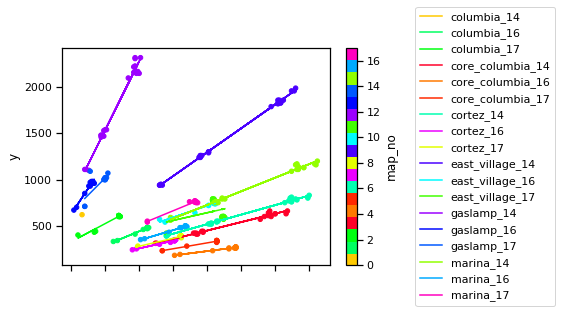Without the constant, it is more clear that the regression lines run through the middle of groups within each map that are linear within the group. For instance, the red line for Cortez, which runs through the sea-foam green points for Cortez, seems to run through two separate groups, one above the regression line, and one below. Other neighborhoods, like columbia,

In :
groups.size()

Out:
map_name          map_no
columbia_14       0          1
columbia_16       1         15
columbia_17       2         15
core_columbia_14  3         23
core_columbia_16  4         15
core_columbia_17  5         15
cortez_14         6         24
cortez_16         7         15
cortez_17         8         15
east_village_14   9         24
east_village_16   10        15
east_village_17   11        26
gaslamp_14        12        23
gaslamp_16        13        15
gaslamp_17        14        15
marina_14         15        24
marina_16         16        15
marina_17         17        15
dtype: int64
In :
groups = dims.groupby(['map_name', 'map_no'])

ax = dims.plot.scatter(x='x',y='y',c='map_no', colormap=cmap)

for (name, num), g in groups:

Y = g.y.to_numpy()
X = g.x.to_numpy()

model = sm.OLS(Y,X)
results = model.fit()
print(name,  results.params)

ax.plot(X, results.predict(X), label=name, color=cmap(num))

plt.legend(loc='center left', bbox_to_anchor=(1.3, 0.5));

columbia_14 1.878787878787879
columbia_16 0.5879914430441483
columbia_17 1.0129378484066898
core_columbia_14 0.3598779944063882
core_columbia_16 0.18118885500072282
core_columbia_17 0.25189403484016193
cortez_14 0.4136180074799075
cortez_16 0.3374025044722717
cortez_17 0.36376267800430295
east_village_14 1.0365958668197477
east_village_16 0.5735955346529538
east_village_17 0.5043044684267859
gaslamp_14 3.052536485289319
gaslamp_16 2.404971024990945
gaslamp_17 2.0565585042572487
marina_14 0.5828126247626086
marina_16 0.45194709546751755
marina_17 0.6563124760729933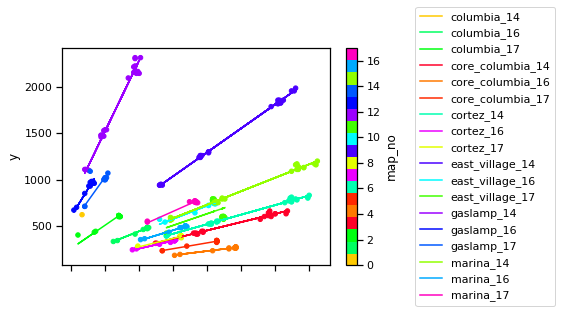## K-Means Clustering¶

The other idea for clustering is k-means. The k-means procedure unfortunately requires knowing how many clusters you want, and we don't know. But, we do nknow it is a small number. So, we can run the process multiple times and look for the number of clusters where the matching score is sufficiently good. The scores will ( should form a plot ) with a knee, which you can find easily with the kneed package

In :
t = dims[dims.map_name == 'gaslamp_16']
X = t[['x','y']].values

scores = []

for i in range(2,10):
kmeans = KMeans(n_clusters=i, random_state=0).fit(X)
pred = kmeans.predict(X)
scores.append(kmeans.score(X))

knee = KneeLocator(x=range(len(scores)), y=scores)

knee.plot_knee();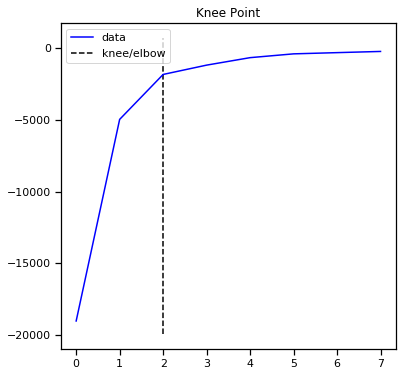Here is the whole procedure to first run multiple k-means to find the optimal clusters, then run it one more time to find the clusters.

In :
##
## Use KMeans to find clusters of region point shapes, to identify different kinds of maps.
##
dims['cluster'] = None

# Force some of the maps to a specific number of clusters.
force_clusters = {
'east_village_c': 2 # Not clear why it finds 4, not 2
}

for name, g in dims.groupby('map_name'):
X = g[['x','y']].values

# If there isn't a lot of variation in the shapes, then skip
# the clustering.
m = X.mean(axis=0) # Centroid

# hmmm .. the distance from the origin to the point?
s = [np.linalg.norm(x-m) for x in X]

if pd.Series(s).std() > 100:

#
# Run the KMeans multiple times and look for the knee in the
# score vs #clusters plot

if not name in force_clusters:
scores = []
n_clusters = []
for i in range(2,10):
kmeans = KMeans(n_clusters=i, random_state=0).fit(X)
pred = kmeans.predict(X)
scores.append(kmeans.score(X))
n_clusters.append(i)

knee = KneeLocator(x=range(len(scores)), y=scores).knee
clusters = n_clusters[knee]
else:
clusters = force_clusters[name]

# Now re-run with that number of clusters.

kmeans = KMeans(n_clusters=clusters, random_state=0).fit(X)
pred = kmeans.predict(X)
else:
print('Skipping ', name)
pred = 0

dims.loc[dims.map_name==name,'cluster'] = pred


Skipping  columbia_14
Skipping  columbia_16
Skipping  columbia_17

/Users/eric/anaconda3/envs/metatab-dev/lib/python3.7/site-packages/sklearn/cluster/k_means_.py:969: ConvergenceWarning: Number of distinct clusters (8) found smaller than n_clusters (9). Possibly due to duplicate points in X.
return_n_iter=True)

Skipping  core_columbia_17
Skipping  cortez_16
Skipping  cortez_17

/Users/eric/anaconda3/envs/metatab-dev/lib/python3.7/site-packages/sklearn/cluster/k_means_.py:969: ConvergenceWarning: Number of distinct clusters (8) found smaller than n_clusters (9). Possibly due to duplicate points in X.
return_n_iter=True)

Skipping  east_village_17
Skipping  gaslamp_16
Skipping  gaslamp_17
Skipping  marina_16

/Users/eric/anaconda3/envs/metatab-dev/lib/python3.7/site-packages/sklearn/cluster/k_means_.py:969: ConvergenceWarning: Number of distinct clusters (8) found smaller than n_clusters (9). Possibly due to duplicate points in X.
return_n_iter=True)

In :
dims['map_cluster'] = dims.apply(lambda r: f"{r.map_name}-{r.cluster}", axis=1)
dims['map_cluster_no'] = dims.map_cluster.astype('category').cat.codes
dims.plot.scatter(x='x',y='y',c='map_cluster_no', colormap='tab20c');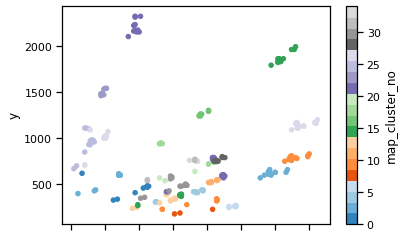That seemed to work, so let's look at the regression again, this time on the clusters, and ignoring the y-intercept.

In :
groups = dims.groupby(['map_cluster', 'map_cluster_no'])

cmap = resample_reorder_cmap(base_cmap,len(groups)) # rand_cmap(len(groups), type='bright')

ax = dims.plot.scatter(x='x',y='y',c='map_cluster_no', colormap=cmap)

import statsmodels.api as sm
for (name, num), g in groups:
Y = g.y.to_numpy()
X = g.x.to_numpy()

model = sm.OLS(Y,X)
results = model.fit()

ax.plot(X, results.predict(X), label=name, color=cmap(num))

plt.legend(loc='center left', bbox_to_anchor=(1.3, 0.5));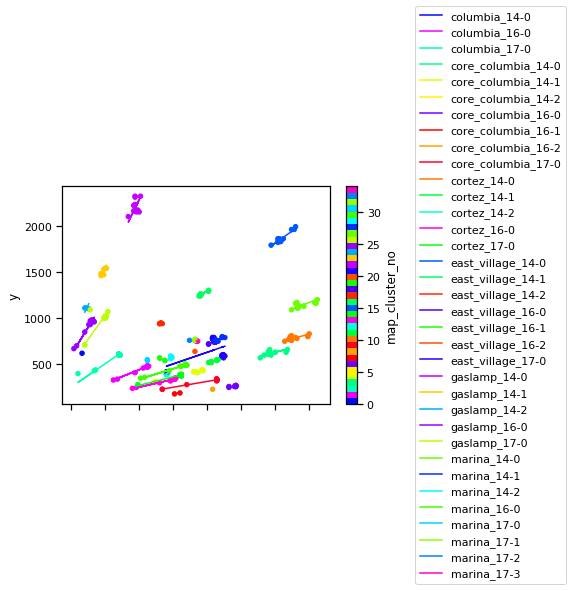These clusters look very good, mostly organized around the regression lines, so we can merge the cluster values back into the main dataset and use the clusters for further analysis.

In :
df = df.merge(dims.reset_index()[['image_url','map_cluster']])

In :
df.head().T

Out:
0 1 2 3 4
image_url http://ds.civicknowledge.org/downtownsandiego.... http://ds.civicknowledge.org/downtownsandiego.... http://ds.civicknowledge.org/downtownsandiego.... http://ds.civicknowledge.org/downtownsandiego.... http://ds.civicknowledge.org/downtownsandiego....
neighborhood columbia columbia columbia columbia columbia
year 2015 2016 2016 2016 2016
month 9 1 2 3 4
intersection_group a a a a a
map_name columbia_14 columbia_16 columbia_16 columbia_16 columbia_16
source_inv POLYGON ((262 1743, 262.5 1123, 585.5 1118.5, ... POLYGON ((832.5 1161, 827.5 753.5, 1541.5 750.... POLYGON ((605 1426.5, 602 1086, 1188 1086, 118... POLYGON ((844.5 1227.5, 848.5 777.5, 1626.5 77... POLYGON ((627 1423, 626 1097.5, 1186.5 1095.5,...
source POLYGON ((262 257, 262.5 877, 585.5 881.5, 590... POLYGON ((832.5 839, 827.5 1246.5, 1541.5 1249... POLYGON ((605 573.5, 602 914, 1188 914, 1185 5... POLYGON ((844.5 772.5, 848.5 1222.5, 1626.5 12... POLYGON ((627 577, 626 902.5, 1186.5 904.5, 11...
source_area 202299 291446 197346 352782 182724
source_shape (330.0, 620.0) (720.0, 410.0) (590.0, 340.0) (780.0, 460.0) (560.0, 330.0)
source_shape_x 330 720 590 780 560
source_shape_y 620 410 340 460 330
dest POLYGON ((6278946 1841436.99999983, 6278953.17... POLYGON ((6278946 1841436.99999983, 6278953.17... POLYGON ((6278946 1841436.99999983, 6278953.17... POLYGON ((6278946 1841436.99999983, 6278953.17... POLYGON ((6278946 1841436.99999983, 6278953.17...
matrix [[2.532279144233197, 0.003906776153235504, 0.0... [[1.1569821727363658, -0.00034090625478441636,... [[1.4149828534968452, 0.0026550014295647142, 0... [[1.0569914364795376, 0.0030690793601490965, 0... [[1.4718082874478764, 0.00020322881428490105, ...
map_cluster columbia_14-0 columbia_16-0 columbia_16-0 columbia_16-0 columbia_16-0

Here are the first images, and the intersections polygon, from one image from each of the clusters.

## Template Matching¶

In :
plot_image_and_poly(df.groupby('map_cluster').first(), max_x=3, figsize=(20,60),
titlef=lambda r: r.map_name)